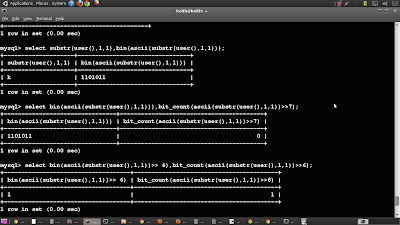### Bit shifting blind injection : Simplified!

I've recently been investigating Blind SQL injection, and have become quite fond of the practice. I stumbled upon a new technique documented by http://h.ackack.net (see here http://h.ackack.net/faster-blind-mysql-injection-using-bit-shifting.html) that used Bit shifting to guess the bits that make up the chars of the information you are trying to extract from the database.

I then came up with a modification to the method to try and make it simpler and hopefully allow the development of an even faster method (which im still working on) . My method uses the XOR bitwise operation to simplify the output and operation of the attack.

Thought relatively simple methods, they still require a comfortable understanding of the binary number system and can be frustrating to use. The method I'm about to show you can be performed with minimal understanding of the binary number system. This is because you don't need to convert in and out of binary while performing the attack.

The Bit counting attack
This method is build on the other attacks but includes another operation that makes everything a lot simpler by counting the TRUE (bits set to 1) bits after every shift is performed.

MySQL has a very convenient function called BIT_COUNT which counts the amount of bits set to 1 in any number given to it. How does this benefit the bit shifting method? Well instead of checking whether a 1 or 0 was added after every shift you could just count the amount of 1s in the binary representation of the number after every shift. This is how you use it:shifting the letter ksome more shifting
What we are doing is counting the number of bits that are set to 1 starting from the left ---if you want you can count from the right too, while shifting another bit out 1 by 1 and checking whether the total number of bits set to 1 increases after every request,
lets say for example we are guessing the the letter 'k' which is 107 in decimal and
1101011 in binary (its also palindromic and prime in binary!! my favourite kind of number)

• 1. bit_count(ascii(substring(user(),1,1))>>6)=1 ---checking if the total amount of 1s is 1, basically we are asking if the left most bit is 1
• if this returns TRUE it means the first bit is a 1, because the total will be 1 and the number will so far look like this: 1??????
• if this returns FALSE it means the first left most bit is a 0 and the number will look like this so far: 0??????
• 2. bit_count(ascii(substring(user(),1,1))>>5)=2 ---checking if the total will increase to 2 bits set to 1 after shifting out one less bit,
• if this returns TRUE it means the second left most bit is a 1, since it will have increased the total count, and the number will look like this: 11?????
• if this returns FALSE it means the second left most bit is a 0, in this case the request will return TRUE
• What do we do when we guess an increase but it returns false? It means we've unravelled a 0 in the number, we then guess the same bit count on the next request, read on to see what I mean
• 3. bit_count(ascii(substring(user(),1,1))>>4)=3 --we guess a 3 here to check if the bits count increases
• if this returns TRUE it means the number is now 111????
• if this returns FALSE it means the number is now 110???? in our case it will return false, so what we do then is still guess the same bit total on the next request hoping the next number is a 0 again
Thats basically it, you do this for a total of 7 times and you'll get the whole number in binary! We did'nt do any converting did we? Told'cha so!! lols

But wait there's more!! There is an even simpler and straight to the point method one can use to get the bits that make up the number, and it too will only cost you 7 requests per character!

Bit sub-string attack
This is crazy I've never been more fascinated by an attack method before
The SUBSTR method isn't limited to just strings, we can pass it binary numbers (this has more to do with MySQL's type handling though)!! So very simply this means in effect you don't hafto do any math while performing the attack!! A Miracle has happened! lols
All we do now is convert the character to binary and pass it to the substring method, then peek at every char in the binary number one by one. This is to be used in a blind attack, so you will have to determine what the page displays as true and what it displays as false (duh!). All you do then is write down a 1 or zero starting from the left depending on if the page returns TRUE(=1) or FALSE(=0). So this is how you use the method.The Bit substring attack
I've only done upto the 3rd, you are to do this up until the 7th bit, after that you have the binary representation of the number

Conclusion
I know it seems quite redundant to remember 4 methods that do the same thing, but in the world of injection hacking its quite handy to be able to perform many variants of the same attack because of the way injection is protected against. I can't imagine any WAF's or Rule sets are prepared to specifically filter out odd functions like BIT_COUNT, so don't write any of the attacks off, master using them all!!

I hope this helped!! Keep hacking guys!! I'll be hitting you with some improvements to this soon i hope :)